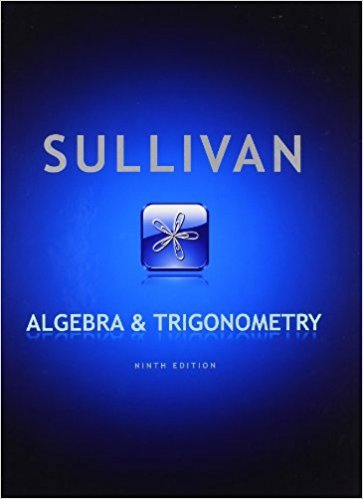×
Log in to StudySoup
Get Full Access to Algebra - Textbook Survival Guide
Join StudySoup for FREE
Get Full Access to Algebra - Textbook Survival Guide

Already have an account? Login here
×
Reset your password

Textbooks / Math / Algebra and Trigonometry 9

# Algebra and Trigonometry 9th Edition - Solutions by Chapter## Full solutions for Algebra and Trigonometry | 9th Edition

ISBN: 9780321716569Algebra and Trigonometry | 9th Edition - Solutions by Chapter

Solutions by Chapter
4 5 0 295 Reviews
##### ISBN: 9780321716569

This expansive textbook survival guide covers the following chapters: 107. This textbook survival guide was created for the textbook: Algebra and Trigonometry, edition: 9. The full step-by-step solution to problem in Algebra and Trigonometry were answered by , our top Math solution expert on 12/23/17, 05:02PM. Since problems from 107 chapters in Algebra and Trigonometry have been answered, more than 96145 students have viewed full step-by-step answer. Algebra and Trigonometry was written by and is associated to the ISBN: 9780321716569.

Key Math Terms and definitions covered in this textbook
• Back substitution.

Upper triangular systems are solved in reverse order Xn to Xl.

• Big formula for n by n determinants.

Det(A) is a sum of n! terms. For each term: Multiply one entry from each row and column of A: rows in order 1, ... , nand column order given by a permutation P. Each of the n! P 's has a + or - sign.

• Characteristic equation det(A - AI) = O.

The n roots are the eigenvalues of A.

• Circulant matrix C.

Constant diagonals wrap around as in cyclic shift S. Every circulant is Col + CIS + ... + Cn_lSn - l . Cx = convolution c * x. Eigenvectors in F.

• Commuting matrices AB = BA.

If diagonalizable, they share n eigenvectors.

• Cramer's Rule for Ax = b.

B j has b replacing column j of A; x j = det B j I det A

• Ellipse (or ellipsoid) x T Ax = 1.

A must be positive definite; the axes of the ellipse are eigenvectors of A, with lengths 1/.JI. (For IIx II = 1 the vectors y = Ax lie on the ellipse IIA-1 yll2 = Y T(AAT)-1 Y = 1 displayed by eigshow; axis lengths ad

• Fibonacci numbers

0,1,1,2,3,5, ... satisfy Fn = Fn-l + Fn- 2 = (A7 -A~)I()q -A2). Growth rate Al = (1 + .J5) 12 is the largest eigenvalue of the Fibonacci matrix [ } A].

• Free columns of A.

Columns without pivots; these are combinations of earlier columns.

• Hilbert matrix hilb(n).

Entries HU = 1/(i + j -1) = Jd X i- 1 xj-1dx. Positive definite but extremely small Amin and large condition number: H is ill-conditioned.

• Hypercube matrix pl.

Row n + 1 counts corners, edges, faces, ... of a cube in Rn.

• Left nullspace N (AT).

Nullspace of AT = "left nullspace" of A because y T A = OT.

• Matrix multiplication AB.

The i, j entry of AB is (row i of A)·(column j of B) = L aikbkj. By columns: Column j of AB = A times column j of B. By rows: row i of A multiplies B. Columns times rows: AB = sum of (column k)(row k). All these equivalent definitions come from the rule that A B times x equals A times B x .

• Network.

A directed graph that has constants Cl, ... , Cm associated with the edges.

• Projection p = a(aTblaTa) onto the line through a.

P = aaT laTa has rank l.

• Random matrix rand(n) or randn(n).

MATLAB creates a matrix with random entries, uniformly distributed on [0 1] for rand and standard normal distribution for randn.

• Reduced row echelon form R = rref(A).

Pivots = 1; zeros above and below pivots; the r nonzero rows of R give a basis for the row space of A.

• Reflection matrix (Householder) Q = I -2uuT.

Unit vector u is reflected to Qu = -u. All x intheplanemirroruTx = o have Qx = x. Notice QT = Q-1 = Q.

• Sum V + W of subs paces.

Space of all (v in V) + (w in W). Direct sum: V n W = to}.

• Symmetric factorizations A = LDLT and A = QAQT.

Signs in A = signs in D.